# Design and Software Implementation of a Navigation Accuracy Evaluation Based on Error Model Solution

Design and Software Implementation of a Navigation Accuracy Evaluation Based on Error Model Solution
Space Control and Inertial Technology Research Center, Harbin Institute of Technology, Harbin 150001, China

Corresponding Author Email:
ygx@hit.edu.cn
Page:
267-273
|
DOI:
https://doi.org/10.18280/i2m.180306
12 February 2019
|
Accepted:
28 May 2019
|
Published:
30 August 2019
| Citation

OPEN ACCESS

Abstract:

This paper attempts to improve the accuracy of navigation accuracy evaluation of inertial navigation system (INS). Firstly, the course effect error of the INS was analyzed in details. Then, the errors of inertial devices like gyro and accelerometer were modelled separately. Based on these models, the author simulated how the course effect error and the second-order errors related to the specific force (SF2Es) affect the speed error, position error and attitude error of the INS, under two trajectory conditions. Based on the solution of the models, a navigation accuracy evaluation software was developed, which can evaluate one or more INSs at the same time. The research findings lay the theoretical basis for INS navigation accuracy evaluation.

Keywords:

gyro, accelerometer, course effect, the second-order error related to the specific force (SF2E), navigation accuracy

1. Introduction

To achieve accurate navigation solution, the inertial navigation system (INS) needs to be corrected and compensated to minimize system error. The error compensation depends on the accuracy of the error model. In fact, the error model is a function of the errors of inertial devices. The more accurate the error model, the better the inertial devices perform, and the more accurate the INS [1, 2]. Currently, there are two ways to establish the error model of inertial device: (1) in-depth analysis of the error sources of inertial devices, and calibration of error coefficients related to temperature, acceleration and angular velocity; (2) statistical analysis of the input-output relationships of inertial devices. The two methods should be selected according to the actual situation [3, 4].

The various errors in the INS can be divided into gyro errors, accelerometer errors, mounting errors of other inertial devices, initial state error of the system, alignment error, etc. The coupling of these errors directly affects the navigation accuracy of the INS . The effect varies with the environments . Among them, the course effect error cannot be neglected in platform inertial navigation system (PINS), due to its significant impact on the navigation accuracy .

Since the platform has a much larger drift angular rate than the gyro, a drift component emerges that changes with the course angle of the platform. Besides, the number of pulses outputted by the gyro under significant heading change differs greatly from that computed based on the calibration results under slight heading change. These phenomena are referred to as the course effect . Repeated experiments have shown that new drift occurs under additional disturbance torque. This drift component is known as the course effect error of the gyro . To calibrate the gyro course effect of the INS, Tang et al.  sets up a mathematical model to analyze the error transmission law, and applies a torque to the gyro torquer for platform precession, aiming to offset the platform course effect and further improve the navigation accuracy.

Many scholars have explained the mechanism of the course effect and compensated the heading drift. For example, Xu et al.  suggests that the gyro needs to move along different paths to the same azimuth from different initial azimuths (i.e. different relative rotation angles between the casing and the platform), and then derives the output variation of the gyro in the same direction. The output variation is mainly because of the difference between the casing and the platform in relative displacement, revealing that the course effect has something to do with the relative movement between the causing and the platform. In addition, the constant drift will change with the additional disturbance torque, when the aircraft changes its heading. Hu and Du  points out that the horizontal two-axis course effect may occur under the zero position of the platform servo circuit or the interference torque on the frame and the frame axis, and analyzes the mechanism of the HE. Moreover, the platform course effect was compensated with the orthogonal elastic drift compensation circuit, reducing the drift by 90 %. He et al.  highlights the fatal effect of the course effect on high-precision space-stable PINS, examines the interaction between heading change, temperature and platform drift variation, and proposes to compensate the course effect error with the temperature-varying Fourier expansion. Xu et al.  explores the influence mechanism of under the zero position of the platform servo circuit, the interference torque on the frame axis and the vibrations in the INS over the HE.

The above studies have explained the course effect mechanism and compensated the course effect error. However, there is no report to consider the influence of the course effect over navigation accuracy in maneuvering flight, or the impacts of the second-order error related to the specific force (SF2E) on the navigation accuracy under different trajectory conditions. To make up for these gaps, this paper explores how the navigation accuracy is affected by the course effect and the SF2E under different environments, improves the error model for the evaluation of navigation accuracy, and designs an evaluation software for the INS navigation accuracy.

2. Course Effect Error

The course effect exerts different degrees of impacts on different platforms. The most significant impact appears on the dynamically tuned gyro platform, which reaches 0.1~0.2°/h in amplitude. The platform will select a maneuvering strategy for orbital transfer that best suits the target. Each strategy is unique in casing-platform angle and platform drift features, call for a specific method to calibrate and compensate errors.

The course effect error has good repeatability, and varies with the platform frame angles. The course effect error should be calibrated regularly, such that the errors could be corrected in real time. The course effect error could be calibrated passively, taking the turntable as the reference of azimuth measurement. Passive calibration is usually performed in the lab. The INS must be removed from the missile and installed on the turntable for calibration. This complex calibration method consumes a long time and raises a strict requirement on the test site.

The course effect error could also be calibrated actively in any site, without using the turntable. The active calibration utilizes the structural features of the INS framework: the rotating platform moves relatively to the framework, changing the course angle. Thus, the changing course angle is measured to compute the drift output of the test point. On the upside, the active method supports self-calibration on the missile and enjoys a wide application range. On the downside, the method takes a long time for readjustment and faces a large interference. During active calibration, manual commands are applied on the platform azimuth, such that the platform moves stably relative to the casing. Then, the course sensors will measure and record data from 6 or 8 reference test points.

The data of active calibration can be extracted in two methods, namely, the course effect error method and rotating casing method. The former is cited to explain the data extraction of active calibration. The extraction of course effect test data is complicated by the fact that the angular velocity component of the earth's rotation is not a constant, when the azimuth angle changes. In the z-axis direction, ${{\varepsilon }_{i{{e}_{z}}}}={{\omega }_{ie}}\sin \varphi$ does not change with the azimuth angle. Thus, the two extraction methods are the same. Along the x- and y- axes, the torque currents of ${{\varepsilon }_{i{{e}_{y}}}}={{\omega }_{ie}}\cos \varphi \cos \alpha$ and ${{\varepsilon }_{i{{e}_{x}}}}={{\omega }_{ie}}\cos \varphi \sin \alpha$ change with the azimuth angle. Therefore, the two components need to be computed. Taking the y-axis for instance, the angular velocity component of the earth’s rotation at each measuring point can be expressed as:

$\left\{ \begin{matrix} {{\varepsilon }_{ie{{y}_{0}}}}={{\omega }_{ie}}\cos \varphi \cos \alpha \\ {{\varepsilon }_{ie{{y}_{1}}}}={{\omega }_{ie}}\cos \varphi \cos \left( \alpha +1\times {{360}^{{}^\circ }}/n \right) \\ {{\varepsilon }_{ie{{y}_{_{2}}}}}={{\omega }_{ie}}\cos \varphi \cos \left( \alpha +2\times {{360}^{{}^\circ }}/n \right) \\ {{\varepsilon }_{ie{{y}_{_{3}}}}}={{\omega }_{ie}}\cos \varphi \cos \left( \alpha +3\times {{360}^{{}^\circ }}/n \right) \\ \vdots \\ {{\varepsilon }_{ie{{y}_{_{n}}}}}={{\omega }_{ie}}\cos \varphi \cos \left( \alpha +n\times {{360}^{{}^\circ }}/n \right) \\\end{matrix} \right.$    (1)

The course error of the platform at the reference point can be obtained through the two data extraction methods. Considering that the test data are small sample unequal interval data, the sample size was expanded at proper intervals through interpolation. The common interpolation methods include linear interpolation, Lagrange interpolation, spline interpolation [14, 15]. In actual engineering, Lagrange two-point interpolation, Lagrange three-point quadratic interpolation (parabolic interpolation) and least squares approximation should be selected if the number of measuring points and test accuracy need to be considered .

3. Error Models of Inertial Devices

3.1 Gyro error model

The INS errors can be divided into deterministic error and random error. The former can be fully compensated, while the latter can only be described statistically, due to the influence of random disturbance. For example, the scale error of the gyro is a deterministic error . During initial alignment, this error can be compensated using calibration or the technical indices provided by the producer . Meanwhile, the zero error is a complex random error of the gyro, and can only be described as a random process [19-20]. In the PINS, the gyro errors are usually illustrated by the static drift model in the online motion environment:

\begin{align} & {{\omega }_{d}}={{K}_{d}}+{{K}_{x}}{{a}_{z}}+{{K}_{y}}{{a}_{y}}+{{K}_{z}}{{a}_{z}}+{{K}_{xy}}{{a}_{x}}{{a}_{y}} \\ & +{{K}_{yz}}{{a}_{y}}{{a}_{z}}+{{K}_{xx}}a_{x}^{2}+{{K}_{yy}}a_{y}^{2}+{{K}_{zz}}a_{z}^{2} \\\end{align}            (2)

where, ωd is the drift rate error; Kd is the constant drift; Kxy, Kyz and Kxz are cross product errors related to the specific force; Kx, Ky and Kz are the first-order errors related to the specific force; Kxx, Kyy and Kzz are the SF2Es; ax, ay and az are the specific force along the corresponding axis.

3.2 Accelerometer error model

The quartz flexible accelerometer, as a high-precision pendulum sensor accelerometer, is widely seen in modern INSs, thanks to its high accuracy and reliability. There are many error sources of the quartz flexible accelerometer, including but not limited to stability error of scale factor, nonlinear error and zero offset. Our research mainly focuses on zero offset, scale error, mounting error and second-order errors. The zero offset consists of constant zero offset and random zero offset; the second-order errors refer to the output errors related to the square of the input acceleration, i.e. second-order nonlinear coefficients. Based on the above analysis and accelerometer output principle, the accelerometer errors of the PINS can be modelled as:

$\delta f\text{=(}{{S}^{a}}+{{M}^{a}}\text{)}{{k}^{a}}{{({{N}^{a}}+D_{2}^{a}{{({{N}^{a}})}^{2}})}^{2}}+{{\nabla }_{a}}+\delta {{\nabla }_{a}}$    (3)

where, ka is the scale factor measured in the lab; Na is the number of output pulses; ${{S}^{a}}=\left[ \begin{matrix} \delta k_{11}^{a} & 0 & 0 \\ 0 & \delta k_{22}^{a} & 0 \\ 0 & 0 & \delta k_{33}^{a} \\\end{matrix} \right]$is the scale factor; ${{M}^{a}}=\left[ \begin{matrix} 1 & \delta k_{12}^{a} & \delta k_{13}^{a} \\ \delta k_{21}^{a} & 1 & \delta k_{23}^{a} \\ \delta k_{31}^{a} & \delta k_{32}^{a} & 1 \\\end{matrix} \right]$ is the matrix of mounting errors; $D_{2}^{a}=\left[ \begin{matrix} \delta k_{11}^{a} & 0 & 0 \\ 0 & \delta k_{22}^{a} & 0 \\ 0 & 0 & \delta k_{33}^{a} \\\end{matrix} \right]$ is the matrix of second-order errors, with $\delta k_{11}^{a}$, $\delta k_{22}^{a}$ and $\delta k_{33}^{a}$ being the second-order error of each axis; ${{\nabla }_{a}}\text{= }\!\![\!\!\text{ }\begin{matrix} {{\nabla }_{ax}} & {{\nabla }_{ay}} & {{\nabla }_{az}} \\\end{matrix}{{\text{ }\!\!]\!\!\text{ }}^{T}}_{{}}$and $\delta {{\nabla }_{a}}\text{=}{{\left[ \begin{matrix} \delta {{\nabla }_{ax}} & \delta {{\nabla }_{ay}} & \delta {{\nabla }_{az}} \\\end{matrix} \right]}^{\text{T}}}$ are the constant zero-offset and the random zero-offset, respectively, with x, y and z being the three axes.

The following accelerometer errors that might affect the navigation accuracy: scale error, zero-offset errors, mounting error, the errors related to acceleration and the errors related to the square of acceleration.

If the PINS is a static or low dynamic environment, the nonlinear errors of the accelerometer are neglected, and only linear errors need to be modelled. If the PINS is in a dynamic environment, the navigation error induced by the nonlinear errors of the accelerometer will gradually accumulate. If this error is considered, the model solution will be more accurate.

In the static or low dynamic environment, the second-order errors of the accelerometer are often ignored. If the PINS makes multiple turns or speeds up, the SF2Es must be taken into account. Therefore, this paper analyzes the excitation of the SF2Es under the dynamic environment. The other errors, which may also be excited in such an environment, are not considered due to the lack of time.

4. Simulation Verification

Two trajectories were set up on an aircraft trajectory design software, namely, the trajectory without maneuvering flight and the trajectory with maneuvering flight (Figure 1).

## 1.png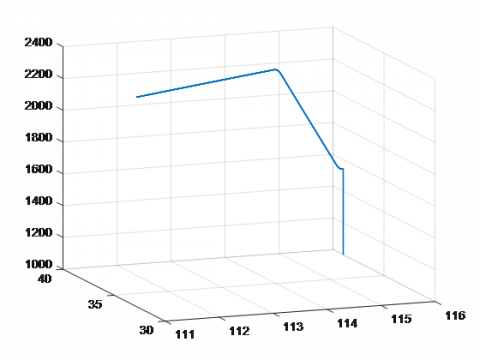(a) The trajectory without maneuvering flight

## 1b.png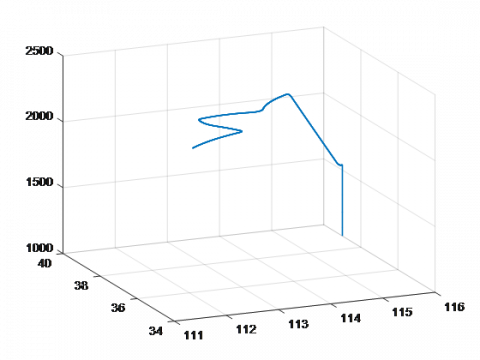(b) The trajectory with maneuvering flight

Figure 1. The trajectories of an aircraft

4.1 Effects of course effect error on navigation accuracy

The INS error model was used to simulate the navigation accuracy under course effect error without maneuvering flight. The results (red solid curves) were compared with those without course effect error (black dotted curves) (Figures 2~4).

## 2.png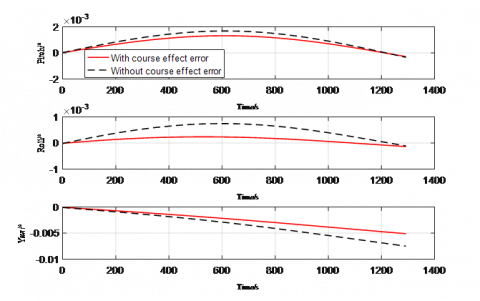Figure 2. Effects of course effect error on navigation attitude (without maneuvering flight)

## 3.pngFigure 3. Effects of course effect error on navigation speed (without maneuvering flight)

From Figures 2~4, it can be seen that, without maneuvering flight, the navigation accuracy in the presence of course effect error was always better than that in the absence of course effect error, revealing the significant impacts of course effect error on navigation accuracy without maneuvering flight.

Next, the navigation accuracy was simulated under course effect error with maneuvering flight. The results (red solid curves) were compared with those without course effect error (black dotted curves) (Figures 5~7). Note that the evaluation accuracy was increased by 5 %.

As shown in Figures 5~7, with maneuvering flight, the navigation accuracy in the presence of course effect error was always better than that in the absence of course effect error. Therefore, the course effect error exerts major impacts on navigation accuracy, with or without maneuvering flight.

## 4.png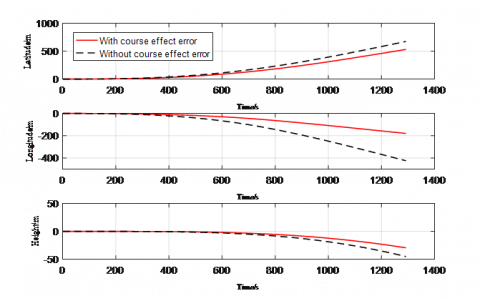Figure 4. Effects of course effect error on navigation position (without maneuvering flight)

## 5.png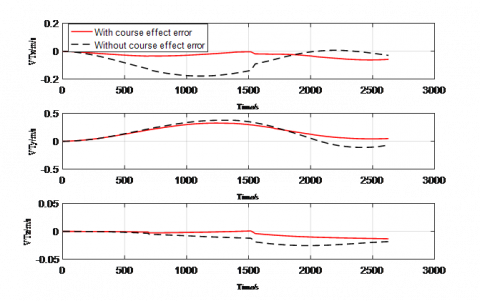Figure 5. Effects of course effect error on navigation attitude (with maneuvering flight)

## 6.png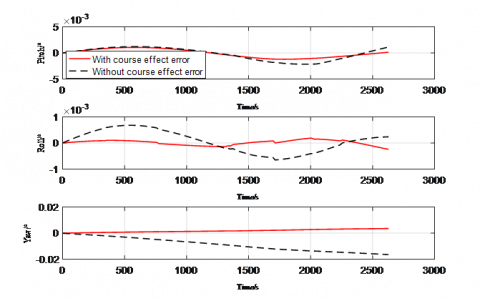Figure 6. Effects of course effect error on navigation speed (with maneuvering flight)

## 7.png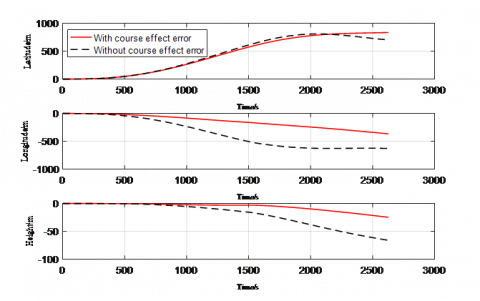Figure 7. Effects of course effect error on navigation position (with maneuvering flight)

4.2 Effects of the SF2Es on navigation accuracy

The effects of the SF2Es were simulated with the following parameters: the gyro’s SF2E, 0.05°/h/g2; the accelerometer’s SF2E, 5×10-5s2/m.

Without considering the other errors (e.g. course effect error), the effects of the SF2Es on navigation accuracy were simulated without maneuvering flight. The results (red solid curves) were compared with those without considering the SF2Es (black dotted curves) (Figures 8~10).

## 8.pngFigure 8. Effects of the SF2Es on navigation attitude (without maneuvering flight)

## 9.pngFigure 9. Effects of the SF2Es on navigation speed (without maneuvering flight)

## 10.png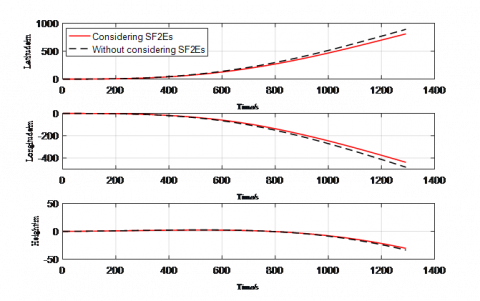Figure 10. Effects of the SF2Es on navigation position (without maneuvering flight)

It can be seen from Figures 8~10 that, without maneuvering flight, the navigation accuracy remained basically the same, whether the SF2Es are considered or not. More careful observations show that the navigation accuracy considering the SF2Es was slightly better than that without considering the SF2Es. Thus, the SF2Es have a negligible impact on navigation accuracy.

Considering the other errors (e.g. course effect error), the effects of the SF2Es on navigation accuracy were simulated with maneuvering flight. The results (red solid curves) were compared with those without considering the SF2Es (black dotted curves) (Figures 11~13). Note that the accelerometer SF2E was adjusted to 1×10-5s2/m.

## 11.pngFigure 11. Effects of the SF2Es on navigation attitude (with maneuvering flight)

## 12.pngFigure 12. Effects of the SF2Es on navigation speed (with maneuvering flight)

## 13.pngFigure 13. Effects of the SF2Es on navigation position (with maneuvering flight)

As shown in Figures 10~13, with maneuvering flight, the navigation accuracy considering the SF2Es was clearly better than that without considering the SF2Es. This means the SF2Es have significant impacts on navigation accuracy with maneuvering flight. To sum up, the SF2Es can be neglected without maneuvering flight, but must be considered with maneuvering flight.

5. Software Design and Implementation

This section designs a navigation accuracy evaluation software based on error model solution. The software interface is shown in Figure 15. The navigation accuracy is evaluated in the following steps: First, click on the lower left button to upload the trajectory. Then, input the errors of inertial devices at the center of the interface, and configure the errors. After that, select “number of sampling collections” and “classification threshold” in the lower middle part. The number of sampling collections stands for the number of iterations to evaluate the navigation accuracy, while the classification threshold varies with the PINS level (the PINSs with different functions require different navigation systems). In the interface, the upper right corner lists the impact points and the circles representing the levels of evaluated navigation accuracy; the lower right part is the map of x and y-axis speed errors. To determine the navigation accuracy of the PINS, the evaluation results in the lower right part should be evaluated against the preset indices.

## 14.jpg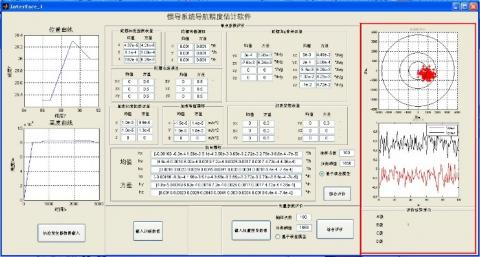Figure 14. Interface of navigation accuracy evaluation software

After solving a single PINS error model, the author inputted the trajectory and inertial device errors, and set and the number of sample collections and classification threshold to 100 and 1n mile, respectively. The samples were collected by Monte-Carlo method. Four levels of navigation accuracy were defined: Class A (the impact point deviates from the target by less than 0.5n mile), Class B (the impact point deviates from the target by 0.5~1n mile), Class C (the impact point deviates from the target by 1~2nm) and Class D (the impact point deviates from the target by greater than 2n mile). The impact points are shown in red boxes of Figure 14. The x- and y-axis speed errors and evaluation results are also given in that figure.

It can be seen that the probabilities for x- and y-axis speeds to fall within ±1m/s were 74.32% and 70.2%, respectively; the probability for the impact point to deviate from the target by less than 1n mile was 84.4%. Therefore, this PINS is a Class B system in terms of navigation accuracy.

The software also offers a window to import INS data in batches, enabling the navigation accuracy evaluation of multiple INSs. The user only needs to select the relevant data and click on the open button. Here, the data of four PINSs are uploaded at the same time. Once the data have been uploaded, a prompt will appear signifying the success of uploading. The number of sample collections and classification threshold were set to 100 and 1,852m, respectively. Then, the author selected error model-based evaluation method in the red box of Figure 16, and clicked on the comprehensive evaluation button. Soon, the evaluation results appeared in that red box.

## 15.jpg## 16.jpgFigure 16. The navigation accuracy evaluation of multiple PINSs

The final impact points of the four PINSs were presented in different colors on the upper right of Figure 16. Red points were the closest to the ideal impact points, followed in turn by black points, blue points and pink points. In the middle of the red box were the x- and y-direction speed errors of the four PINSs. The x-direction speed error was colored black and the y-direction speed error was colored red. The evaluation results on the four PINSs were displayed on the lower right corner: the first PINS belongs to Class B, the second and third PINSs belong to Class C, and the fourth PINS belong to Class D.

6. Conclusions

Starting with the error equations of the INS, this paper analyzes the effects of course effect error on the PINS navigation error, and develops the error models for gyro and accelerometer. Based on these models, the author examined how the course effect error and the SF2Es affect the speed error, position error and attitude error of the INS, under two trajectory conditions. Finally, a navigation accuracy evaluation software was designed and implemented. The software can compute the speed errors and evaluate the level of navigation accuracy of one or more INSs based on the deviation of impacts points from the target.

References

 Gao, X., Bian, H.W., Fan, S.W. (2013). Optimal damping algorithm design in inertial navigation system. 2013 International Conference on Mechatronic Sciences, Electric Engineering and Computer (MEC). https://doi.org/10.1109/MEC.2013.6885534

 Nobahari, H., Mohammadkarimi, H. (2017). Accuracy analysis of an integrated inertial navigation system in slow maneuvers. Navigation- Journal of the Institute of Navigation, 64(3): 197-211. https://doi.org/10.1002/navi.195

 Li, C., Cao, Y., Zhang, S.F. (2015). A new multi-position calibration method for accelerometers of the inertial navigation system. Control & Decision Conference, 6511-6514.

 Hu, Z.D., Zhang, L.X., Zhou, F.Y., Li, Z.J. (2013). Statistic inference for inertial instrumentation error model using Bayesian network. Applied Mechanics and Materials, 392: 719-724. https://doi.org/10.4028/www.scientific.net/amm.392.719

 Filyashkin, N.K., Yatskivsky, V.S. (2013). Prediction of inertial navigation system error dynamics in INS/GPS system. Actual Problems of Unmanned Air Vehicles Developments Proceedings (APUAVD), 2013 IEEE 2nd International Conference. https://doi.org/10.1109/APUAVD.2013.6705327

 Wang, C.F., Wang, H.Y., Shi, Z.S., Zhan, M.F. (2011). Maritime sensor registration algorithm based on high-precision navigation equipment. Control Theory & Applications, 28(4): 497-503.

 Gu, D.Q., El-sheimy, N. (2008). Heading accuracy improvement of MEMS IMU/DGPS integrated navigation system for land vehicle, position, location & navigation symposium. IEEE/ION. https://doi.org/10.1109/PLANS.2008.4570083

 Jiang, Y.G., Guo, L., Jia, Z.Q., Wu, X.X. (2011). Calibration and compensation methods for heading effect of intertial navigation platform in dynamic of TT&C-Ship. Ship Science and Technology, 8168(1): 372-377. https://doi.org/ 10.1117/12.896832

 Gao, P.Y., Li, K., Wang, L., Zhang, Q. (2016). Four-position heading effect calibration algorithm for rotation inertial navigation system based on fiber optic gyro. Optical Engineering, 55(7): 074105. https://doi.org/10.1117/1.OE.55.7.074105

 Tang, C.P., Hu, X.L., Zhou, S.S., Guo, R., He, F., Liu, L., Zhu, L.F., Li, X.J., Wu, S., Zhao, G., Yu, Y., Cao, Y.L. (2016). Improvement of orbit determination accuracy for Beidou navigation satellite system with two-way satellite time frequency transfer. Advances in Space Research, 58(7): 1390-1400. https://doi.org/10.1016/j.asr.2016.06.007

 Xu, J.H., Xiao, Z.L., Qian, P.X., Xiong, T. (2005). Study and calibration of course effect of inertial navigation platform. Journal of Projectile and Arrow Guidance, 25(1): 5-8. https://doi.org/10.3969/j.issn.1673-9728.2005.01.002

 Hu, P.H., Du, Z.L. (2001). Compensation of quadrature spring rate Ampl if ier for platform heading sensitive drift. Journal of Chinese Inertial Technology, 2001(1): 31-35. https://doi.org/10.3969/j.issn.1005-6734.2001.01.006

 He, Q.E., Yang, G.L., Zhang, R., Wu, Q.P. (2017). Compensation method of heading effect for inertial navigation system based on Fourier decomposition of temperature variation. Journal of Chinese Inertial Technology, 25(5): 27-30. https://doi.org/CNKI:SUN:ZGXJ.0.2017-05-005

 Argaud, J.P., Bouriquet, B., De Caso, F., Gong, H., Maday, Y., Mula, O. (2018). Sensor placement in nuclear reactors based on the generalized empirical interpolation method. Journal of Computational Physics, 363: 354-370. https://doi.org/ 10.1016/j.jcp.2018.02.050

 Cai, Q.Z., Yang, G.L., Song, N.F., Yin, H.L., Liu, Y.L. (2016). Analysis and calibration of the gyro bias caused by geomagnetic field in a dual-axis rotational inertial navigation system. Measurement Science and Technology, 27(10): 105001. https://doi.org/10.1088/0957-0233/27/10/105001

 Berriochoa, E., Cachafeiro, A., García-Amor, J.M. (2018). Algorithms, convergence and rate of convergence for an interpolation model between Lagrange and Hermite. Results in Mathematics, 73(1): 40. https://doi.org/ 10.1007/s00025-018-0802-0

 Lawson, W.G., Hansen, J.A. (2004). Implications of stochastic and deterministic filters as ensemble-based data assimilation methods in varying regimes of error growth. Monthly Weather Review, 132(8): 1966-1981. https://doi.org/10.1175/1520-0493(2004)132<1966:iosadf>2.0.co;2

 Wang, K.D., Zhu, T.Q., Qin, Y.J., Jiang, R., Li, Y. (2018). Matching error of the iterative closest contour point algorithm for terrain-aided navigation. Aerospace Science & Technology, 73: 210-222. https://doi.org/10.1016/j.ast.2017.12.010

 Joglekar, H., Venkateswarlu, A. (2006). Gyro scale-factor error and misalignment estimation for a spacecraft. Aiaa/aas Astrodynamics Specialist Conference & Exhibit. https://doi.org/10.2514/6.2004-4860

 Aronowitz, F., Killpatrick, J., Callaghan, S. (1974). Power-dependent correction to the scale factor in the laser gyro. IEEE Journal of Quantum Electronics, 10(2): 201-208. https://doi.org/ 10.1109/jqe.1974.1145792